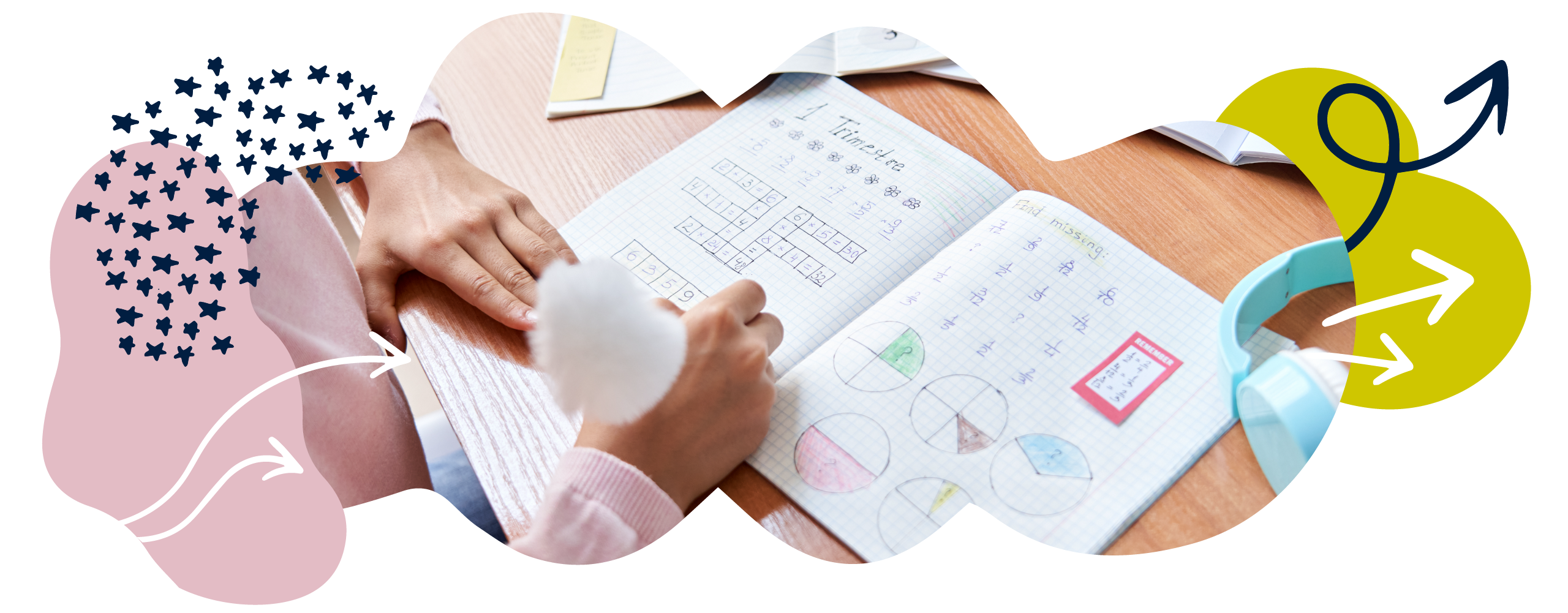We use cookies to enhance your experience on our website. By continuing to use our website, you are agreeing to our use of cookies. You can change your cookie settings at any time. Find out more# Fractions in Year 2 (age 6–7)

In Year 2, your child will continue to learn about halves and quarters. They will also begin to think about thirds.

The key words for this section are equivalence, denominator, numerator, and number line.

## What your child will learn

Take a look at the National Curriculum expectations for fractions in Year 2 (age 6–7):

#### Recognise and use fractions ⅓, ¼, and ¾

Your child will learn how to divide:

• Lengths (for example, a 12cm ribbon).
• Shapes (for example, a rectangle).
• Sets of objects (for example, a toy collection).
• Quantities (for example, 24 sweets).

Your child will divide these into equal groups to find , and  of these numbers.

#### Write simple fractions and recognise equivalences

Your child will use objects to find:

• Halves by sharing equally between two groups.
• Thirds by sharing equally between three groups.
• Quarters by sharing equally between four groups.

They will also be expected to write out fractions in simple calculations. This will help them to understand the connection between fractions and division. For example:

of 6 = 3

6 ÷ 2 = 3

of 12 = 4

12 ÷ 3 = 4

Your child will understand that  is equivalent to  .

## How to help at home

There are lots of everyday ways you can help your child to understand fractions. Here are just a few ideas.

### 1. Exploring fractions of quantities

Try sharing objects equally to find fractions of amounts. You could do this by using things around the house – for example, grapes, buttons, beads, or pieces of dried pasta… the options are endless!

• Halves. Find 12 of the same type of object and ask your child to find half. Ask them how many are in each group and help them see that half of 12 is 6 because there are 6 objects in each group.
• Quarters. Once you’ve explored halves, you could find a quarter of the 12 objects. Show your child that you can do this by sharing the objects into four equal groups or by finding half and then half again.
• Thirds. Now see if your child can share the objects into three equal groups. How many are in each group? Try finding three quarters and then two thirds of the 12 objects.

See if your child understands that two quarters is equivalent to one half, and that four quarters is equivalent to the whole. Help them understand that finding a half is equivalent to dividing by 2, finding a quarter is equivalent to dividing by 4, and finding a third is equivalent to dividing by 3.

### 2. Fractions of shapes

If your child enjoys drawing and crafts, use paper, pens, and scissors to show them how fractions work. Together, draw simple shapes like squares, triangles, or circles. You will need to ensure they are quite even and exact – drawing around things can help with this.

Cut out the shapes and then fold them to find halves, thirds, and quarters of the shapes. Can the shape be folded into two, three, or four equal parts? There are lots of ways you can use these shapes to explore fractions. For example:

Show your child a square and ask them how they would find half of the shape. They may fold the shape in half to see if there are two equal parts. Ask them to show you one half. What shape is the half?

Investigate how many different ways a shape can be folded in half. For instance, you could fold a square diagonally to create triangular shapes. Find different ways to make a quarter of a shape or a third of a shape.

### 3. Writing fractions

Help your child start writing fractions using mathematical symbols. The number at the bottom is called the denominator and the number at the top is called the numerator. The denominator tells us how many equal parts we are dividing into. The numerator tells us how many of the equal parts we are looking for.

You could play a game where children match the written fraction to an image of the fraction. For example:

¼ =### 4. Fractions as numbers in their own right

Your child needs to understand that a fraction is also a number in its own right. You can help your child with this concept using number lines.

You could show your child a number line as shown below. Ask them to show where a half would go on the number line. Help them to understand that a half is directly in the middle of 0 and 1.Similarly, your child will need to be able to find a quarter, three-quarters and a third on a number line, as shown below:### 5. Counting in fractional steps

Encourage your child to begin counting in halves, quarters, and thirds using objects and number lines to help. For example, cut pieces of fruit into three equal pieces and count in thirds: ⅓, ⅔, 1, 1 ⅓, 1 ⅔, and so on.

This will reinforce the idea that fractions are numbers, and will show how they can add up to more than one. Number lines and images can also represent fractions and help you count in thirds. For example: